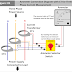Hey, in this article we are going to see the Connection Diagram of a single-phase analog ammeter with a Selector Switch and CTs(Current Transformer) for three-phase current measurement. This type of connection you will see in different types of electrical panels such as power control panels, motor control panels, etc. Ammeter is basically a measuring device that is used to measure the electrical current. A single-phase two-terminal ammeter can be used to measure current flow in a single-phase power system. But by connecting some additional equipment such as a selector switch and CTs we can measure current flow in three phase system also. So, here we will see the whole wiring diagram for current measurement in a three-phase system.

## Ammeter Connection Diagram with Selector Switch and CT

Here, you can see the connection diagram between Ammeter, Selector Switch, and Current Transformers(CTs) for current measurement in a three-phase system.

In this arrangement, you can see the current flow in each phase by rotating the selector switch. When you kept the selector switch in the 'O' position the ammeter will not show any current flow as it is in off condition. When you rotate the switch in the 'R' position the ammeter will show the value of the current flow through the R phase. When you rotate the switch in the 'Y' position the ammeter will show the value of current flow through the B phase. Similarly, when you rotate the switch in the 'B' position the ammeter will show the value of current flow through the B phase.

Connection Procedure
1. First of all, you have to take all the equipment with proper rating. Especially, the current ratio of the CTs and Ammeter must be the same.
2. Now place all the CTs with phase wires between the power circuit and the load circuit and mark the CTs as 1,2, and 3 for R, Y, and B phases respectively.
3. Now connect the phase or positive(anything identified) terminal of the CT1 to the 'R' or 'L1" terminal of the Selector Switch.
4. Connect the phase or positive(anything identified) terminal of the CT2 to the 'Y' or 'L2" terminal of the Selector Switch.
5. Similarly, connect the phase or positive(anything identified) terminal of the CT3 to the 'B' or 'L3" terminal of the Selector Switch.
6. Now connect the neutral terminal of all the CTs together and connect to the 'C' or 'N' terminal of the Selector Switch.
7. Now connect the output terminals of the selector switch(here A1, A2) to the two terminals of the ammeter.
8. After completing all the connections you can turn on the power and operate the selector switch to measure the current.

Understanding CT and Ammeter Current Ratio

For example, if the current ratio of the CT is 100:5. The current flow in the secondary winding(or output terminals) of the CT will be 5A when the actual current flow in the system is 100A. Now, your ammeter should have a current ratio of 5:100. So when the ammeter senses the current 5A it will show 100A on the display. If you take different current ratio for CT and Ammeter, then you have to do more complex calculations for measuring the proper current flow.

Thank you for visiting the Website. Keep visiting for more Updates.

Ammeter Connection Diagram with Selector Switch and CTReviewed by Author on June 09, 2023 Rating: 5